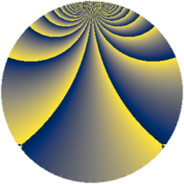# Properties

 Label 900.3.biLevel $900$ Weight $3$ Character orbit 900.bi Rep. character $\chi_{900}(37,\cdot)$ Character field $\Q(\zeta_{20})$ Dimension $200$ Sturm bound $540$

# Related objects

## Defining parameters

 Level: $$N$$ $$=$$ $$900 = 2^{2} \cdot 3^{2} \cdot 5^{2}$$ Weight: $$k$$ $$=$$ $$3$$ Character orbit: $$[\chi]$$ $$=$$ 900.bi (of order $$20$$ and degree $$8$$) Character conductor: $$\operatorname{cond}(\chi)$$ $$=$$ $$25$$ Character field: $$\Q(\zeta_{20})$$ Sturm bound: $$540$$

## Dimensions

The following table gives the dimensions of various subspaces of $$M_{3}(900, [\chi])$$.

Total New Old
Modular forms 2976 200 2776
Cusp forms 2784 200 2584
Eisenstein series 192 0 192

## Trace form

 $$200q + 6q^{5} - 2q^{7} + O(q^{10})$$ $$200q + 6q^{5} - 2q^{7} + 6q^{13} + 32q^{17} - 100q^{19} - 66q^{23} - 80q^{25} - 100q^{29} + 44q^{35} - 6q^{37} + 80q^{41} - 114q^{43} - 158q^{47} - 34q^{53} + 108q^{55} + 250q^{59} - 120q^{61} - 112q^{65} - 90q^{67} + 60q^{71} + 142q^{73} - 100q^{77} + 200q^{79} - 56q^{83} + 368q^{85} + 700q^{89} + 304q^{95} - 50q^{97} + O(q^{100})$$

## Decomposition of $$S_{3}^{\mathrm{new}}(900, [\chi])$$ into newform subspaces

The newforms in this space have not yet been added to the LMFDB.

## Decomposition of $$S_{3}^{\mathrm{old}}(900, [\chi])$$ into lower level spaces

$$S_{3}^{\mathrm{old}}(900, [\chi]) \cong$$ $$S_{3}^{\mathrm{new}}(25, [\chi])$$$$^{\oplus 9}$$$$\oplus$$$$S_{3}^{\mathrm{new}}(50, [\chi])$$$$^{\oplus 6}$$$$\oplus$$$$S_{3}^{\mathrm{new}}(75, [\chi])$$$$^{\oplus 6}$$$$\oplus$$$$S_{3}^{\mathrm{new}}(100, [\chi])$$$$^{\oplus 3}$$$$\oplus$$$$S_{3}^{\mathrm{new}}(150, [\chi])$$$$^{\oplus 4}$$$$\oplus$$$$S_{3}^{\mathrm{new}}(225, [\chi])$$$$^{\oplus 3}$$$$\oplus$$$$S_{3}^{\mathrm{new}}(300, [\chi])$$$$^{\oplus 2}$$$$\oplus$$$$S_{3}^{\mathrm{new}}(450, [\chi])$$$$^{\oplus 2}$$